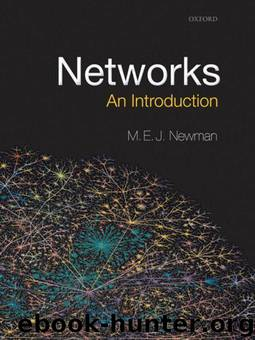# Networks: An Introduction by Newman MarkAuthor:Newman, Mark [Newman, Mark]
Language: eng
Format: mobi
Publisher: Oxford University Press, USA
Published: 2010-05-19T16:00:00+00:00

(12.3)

which is just the standard binomial distribution. Then the mean value of m is

(12.4)

This result comes as no surprise. The expected number of edges between any individual pair of vertices is just equal to the probability p of an edge between the same vertices, and Eq. (12.4) thus says merely that the expected total number of edges in the network is equal to the expected number p between any pair of vertices, multiplied by the number of pairs.

We can use this result to calculate the mean degree of a vertex in the random graph. As pointed out in the previous section, the mean degree in a graph with exactly m edges is 〈k〉 = 2m/n, and hence the mean degree in G(n, p) is